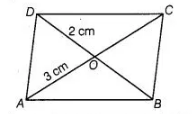# Diagonals AC and BD of a parallelogram`
Question:

Diagonals AC and BD of a parallelogram ABCD intersect each other at O. If OA = 3 cm and OD = 2 cm, determine the lengths of AC and BD.

Solution:

Given, $A B C D$ is a parallelogram $O A=3 \mathrm{~cm}$ and $O D=2 \mathrm{~cm}$We know that, diagonals of a parallelogram bisect each other.

$\therefore \quad$ Diagonal $A C=2 O A=6 \mathrm{~cm} \quad[\because A O=O C]$

and     Diagonal $B D=2 O D=4 \mathrm{~cm}$     $[\because B O=O D]$

Hence, the length of the diagonals $A C$ and $B D$ are $6 \mathrm{~cm}$ and $4 \mathrm{~cm}$, respectively.